# 求解二维浅水波方程的移动网格旋转通量法*

(长安大学 理学院， 西安 710064)

## 1 控 制 方 程

Ut+F(U)x+G(U)y=-S,

(1)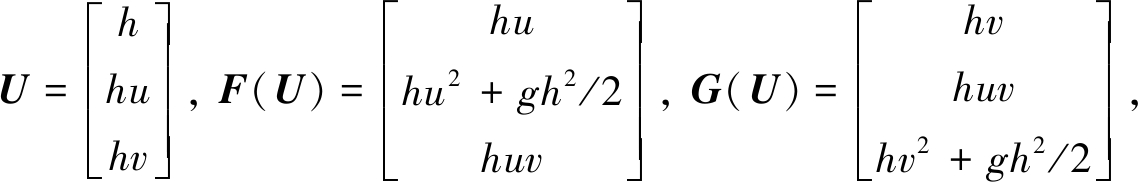h为水深，g为重力加速度，u,v分别为x方向和y方向上的水流速度，S为源项.源项分为摩擦项和倾斜项: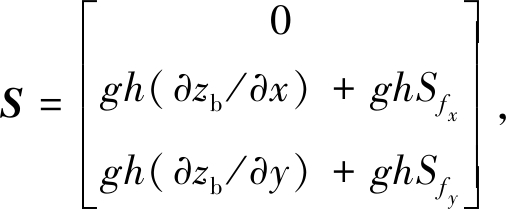(2)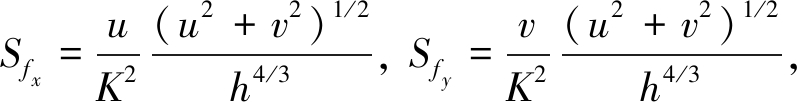(3)

## 2 方 程 离 散

### 2.1 旋转不变性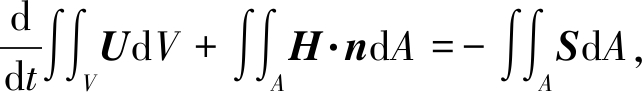(4)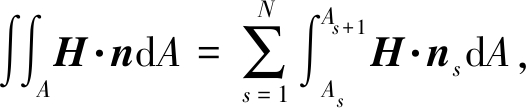(5)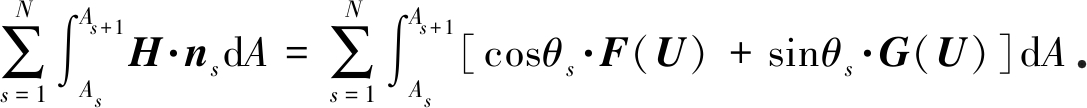(6)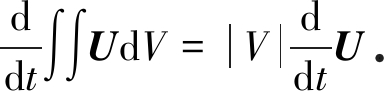(7)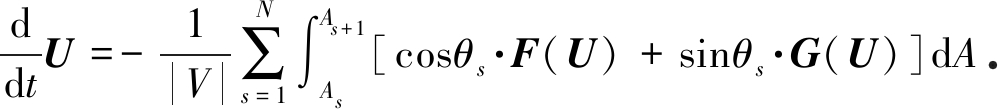(8)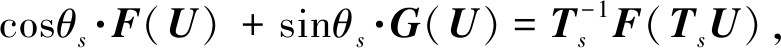(9)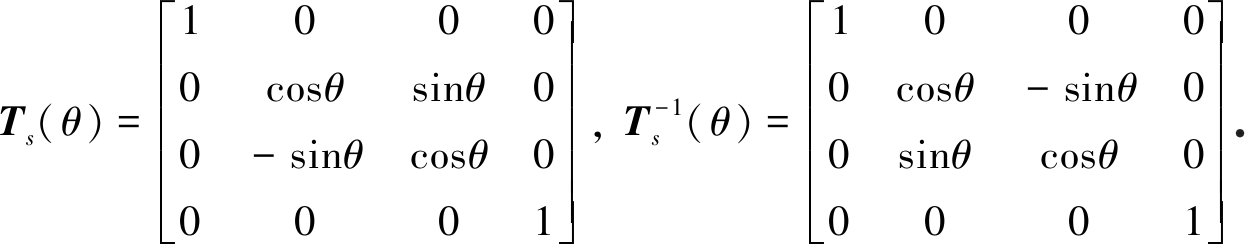(10)

U是旋转守恒通量并且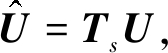将方程(8)变为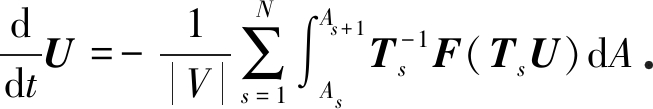(11)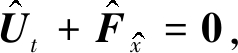(12)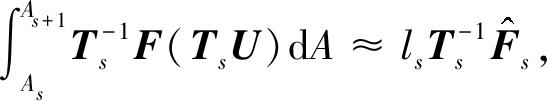(13)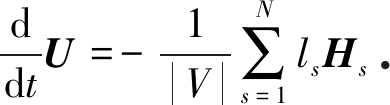(14)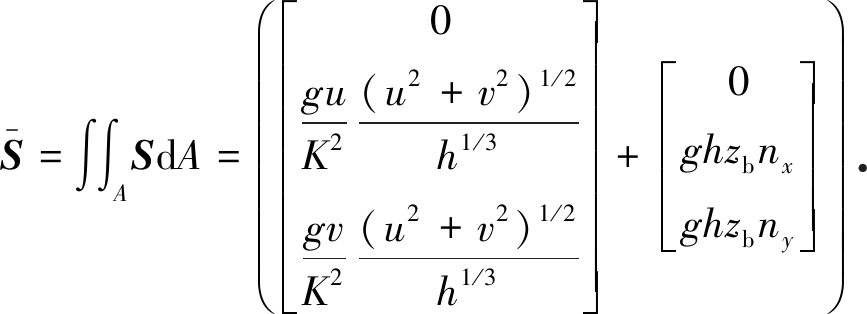(15)

### 2.2 熵稳定格式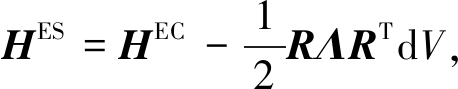(16)

HEC=F·nx+G·ny,

(17)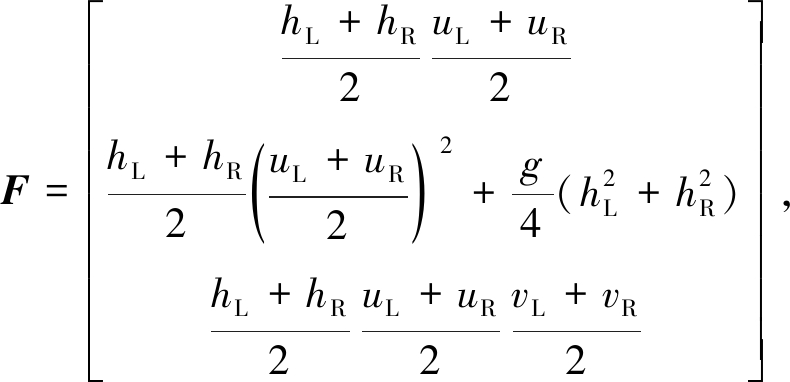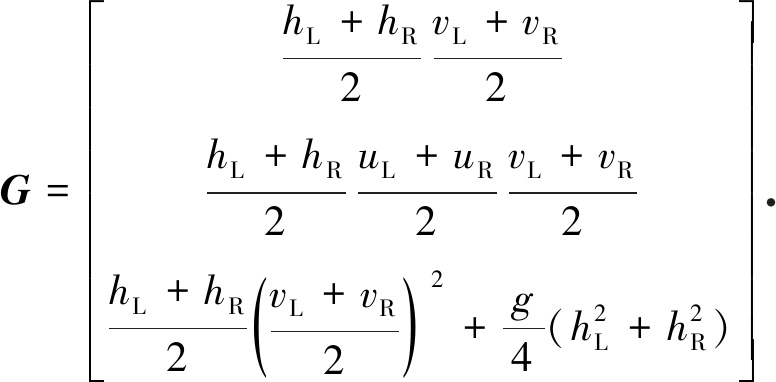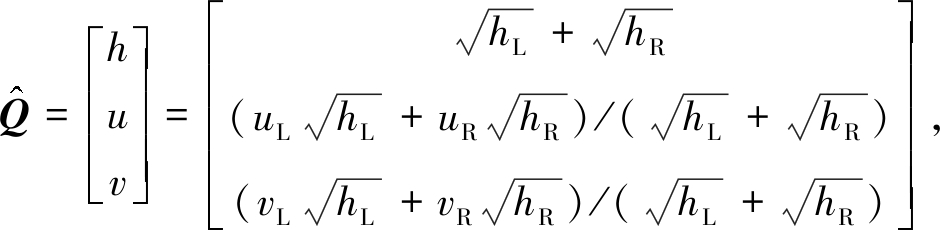(18)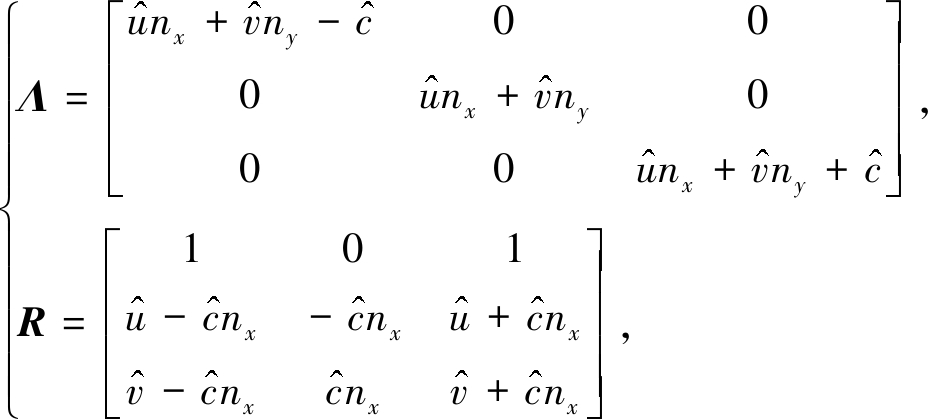(19)

### 2.3 时间离散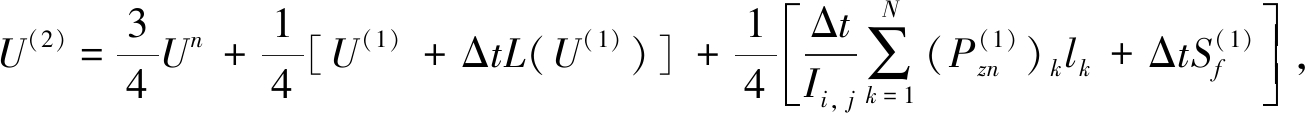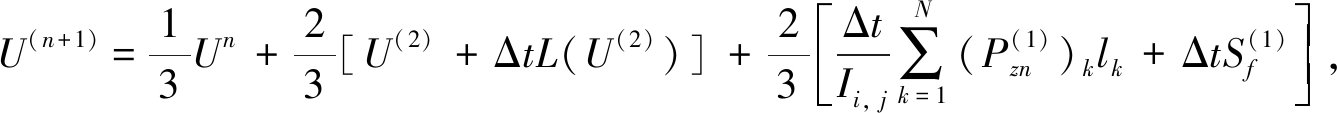## 3 移动网格法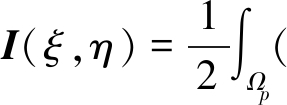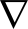ξTG-1ξ+ηTG-1η)dxdy.

(20)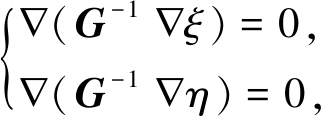(21)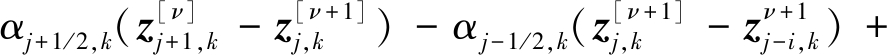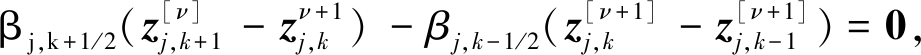(22)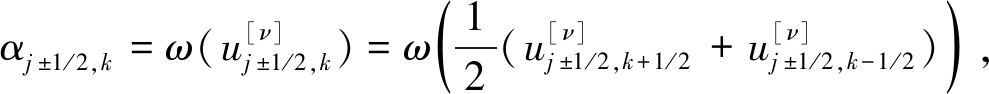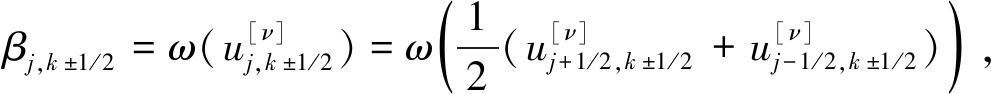ν为迭代步数，一般取3～5步即可.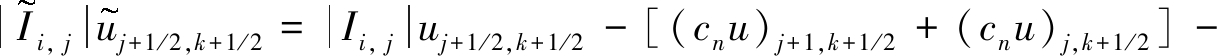[(cnu)j+1/2,k+1+(cnu)j+1/2,k].

(23)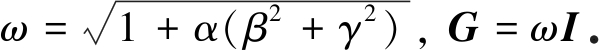(24)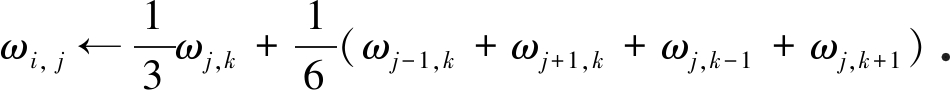(25)

1) 使用等分布原理给定物理域Ωp的初始分区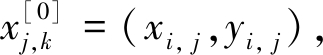使用初始数据u(x,0)计算控制体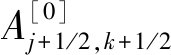上的网格单元均值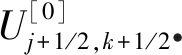2) (a) 网格移动,计算式(21)，将网格点由移动到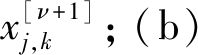守恒变量的更新,用守恒插值式(23)在新控制体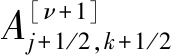上计算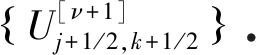3) 在新网格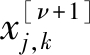上使用高分辨率有限体积法求解浅水波方程，以获得tn+1时刻的近似解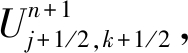空间方向采用熵稳定通量，时间方向采用三阶强稳定的Runge-Kutta方法.

4) 如果tn+1<T,令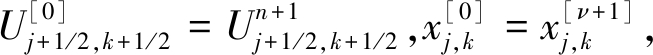返回第2)步直至计算结束.

## 4 数 值 算 例

zb(x,y)=1+0.01cos(πx/2)cos(πy/2),

h(x,y,0)=zb(x,y,0)+ζ(x,y,0), u(x,y,0)=v(x,y,0)=0,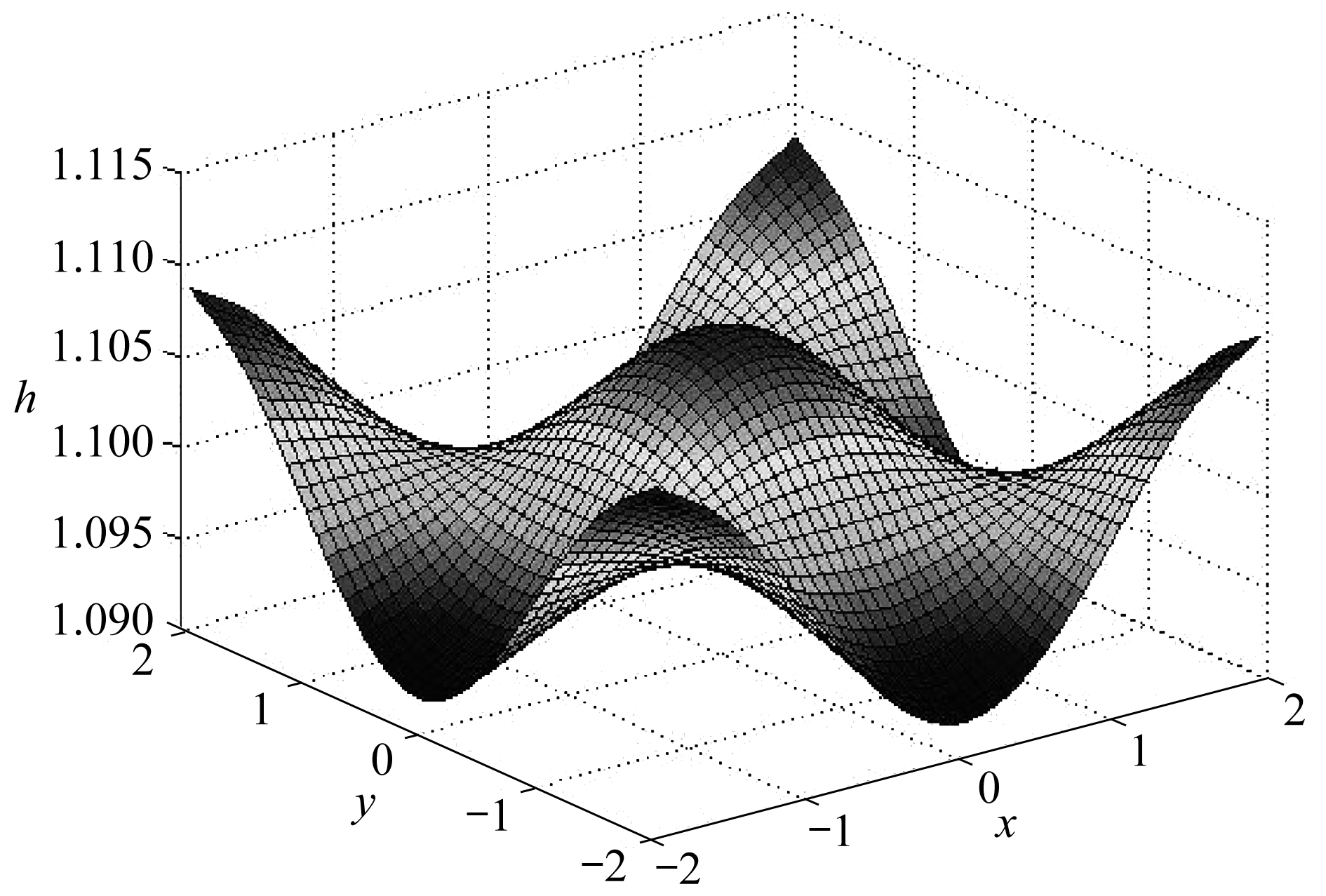Fig. 1 The tide problem simulation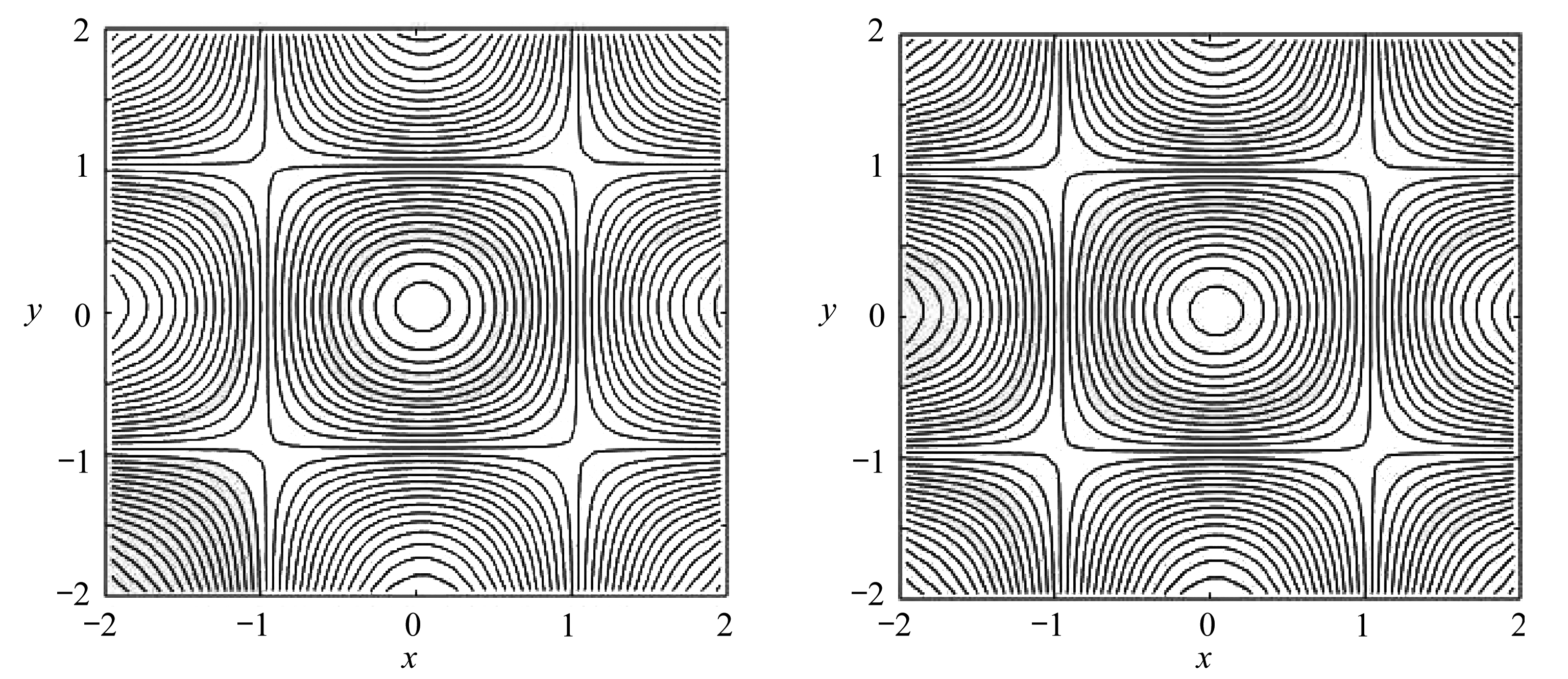(a) 固定网格结果 (b) 移动网格结果 (a) Fixed grid results (b) Moving grid results

Fig. 2 The 2D tide problem water depth contours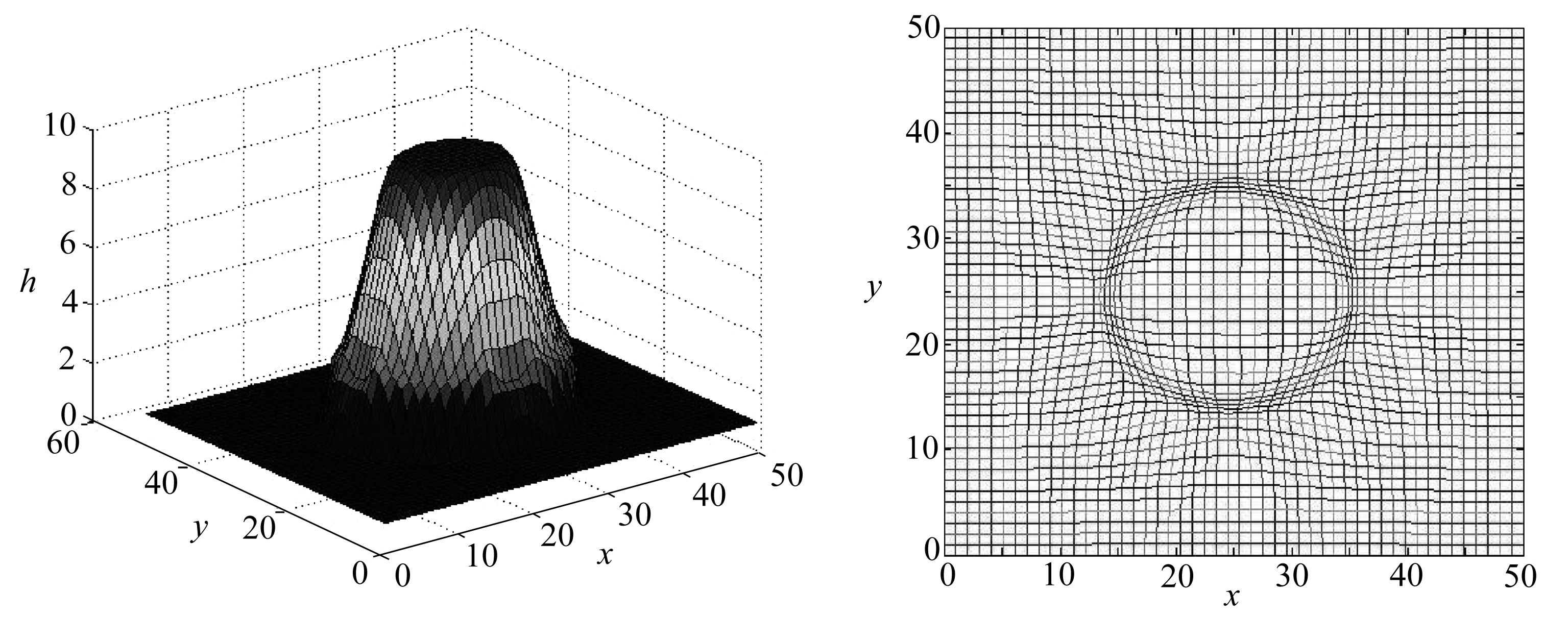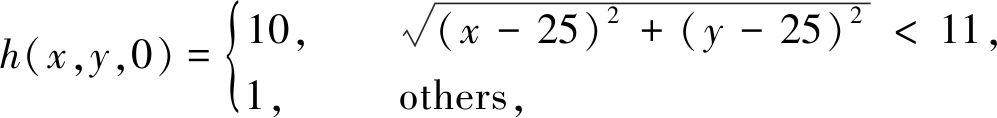u(x,y,0)=v(x,y,0)=0, zb(x,y)=0.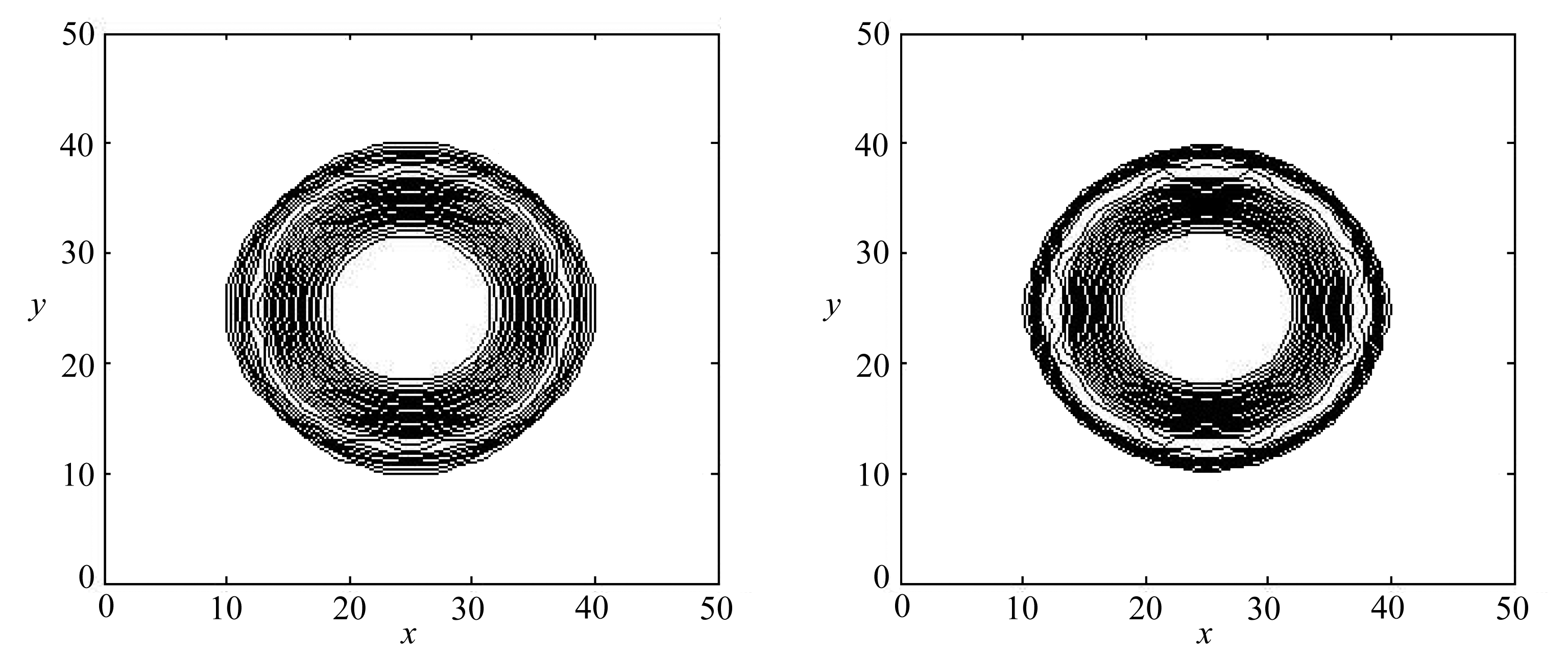(a) 固定网格结果 (b) 移动网格结果 (a) Fixed grid results (b) Moving grid results

Fig. 5 The water depth contours of the 2D cylindrical dam failure problem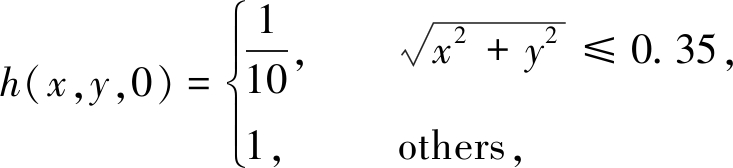u(x,y,0)=v(x,y,0)=0, zb(x,y)=0.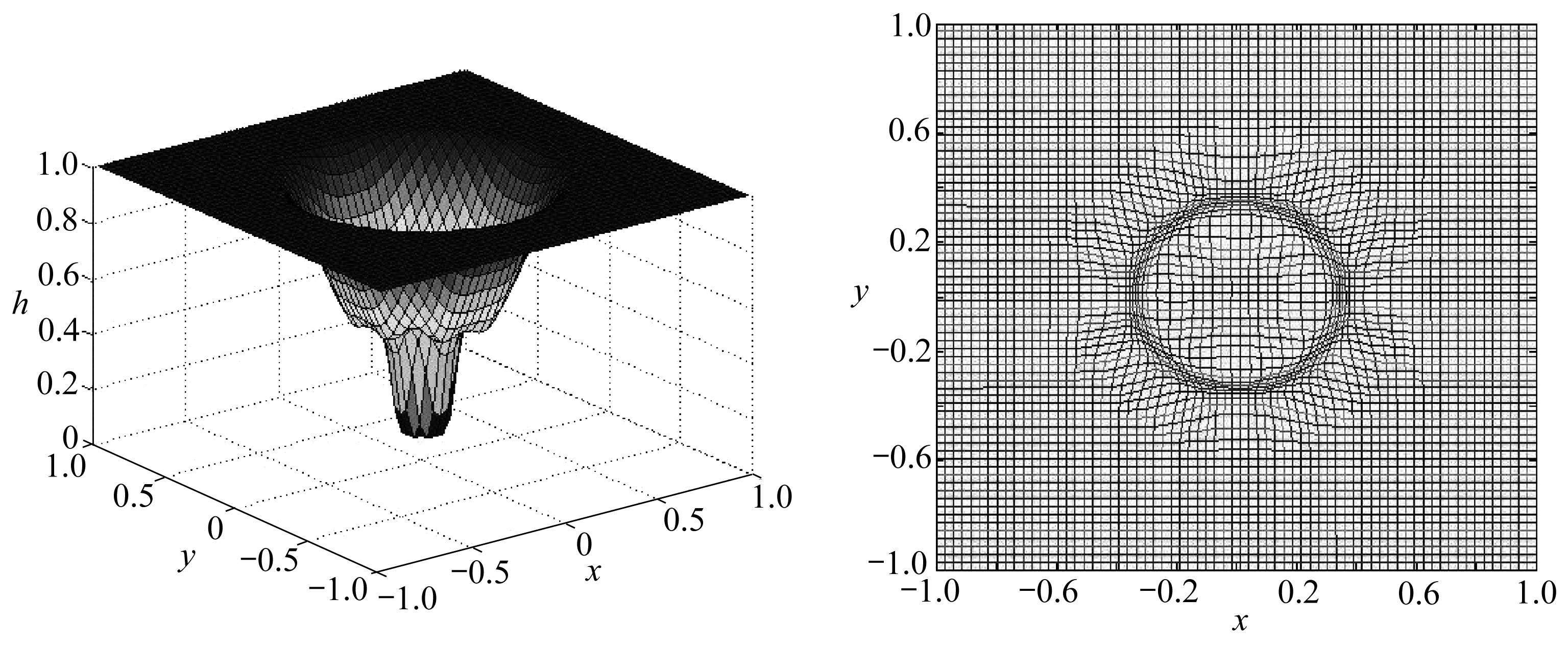Fig. 6 The shock focus simulation Fig. 7 The grid evolution diagram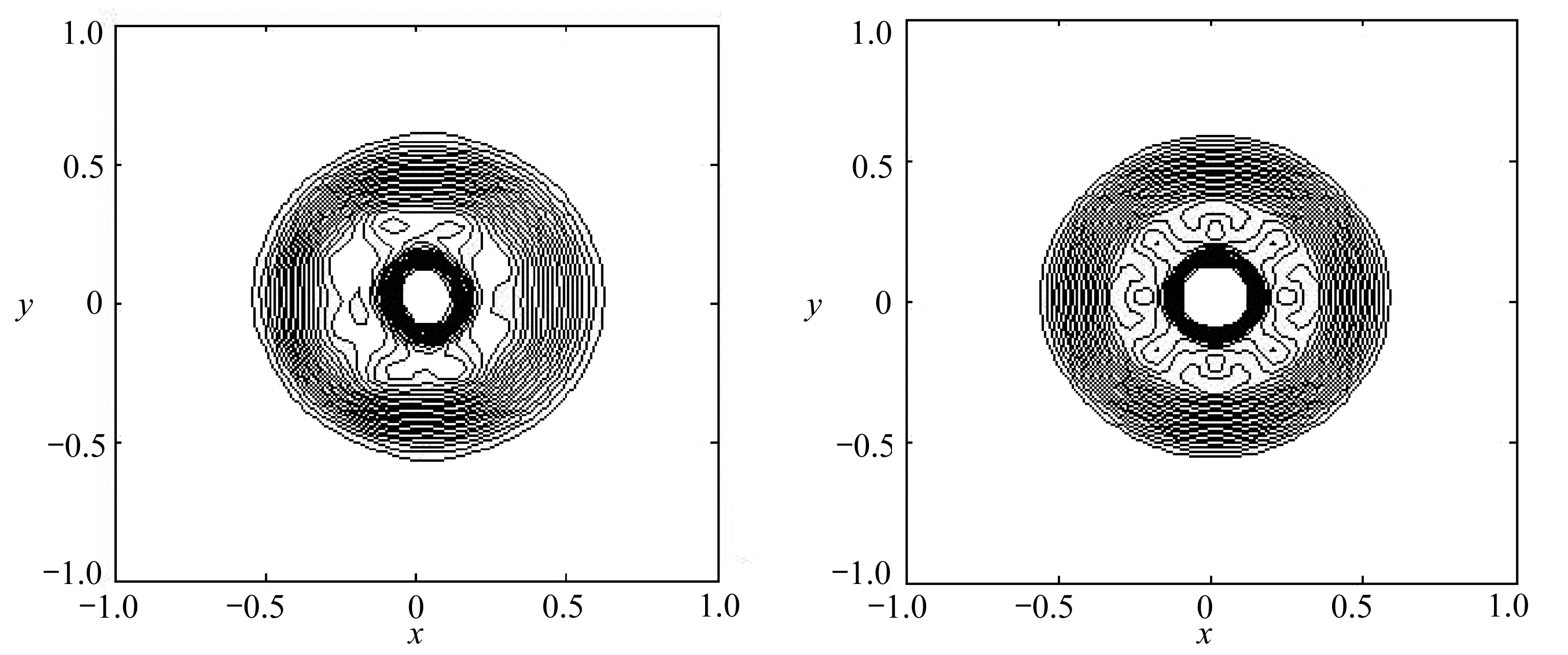(a) 固定网格结果 (b) 移动网格结果 (a) Fixed grid results (b) Moving grid results

Fig. 8 The 2D shock focus problem water depth contours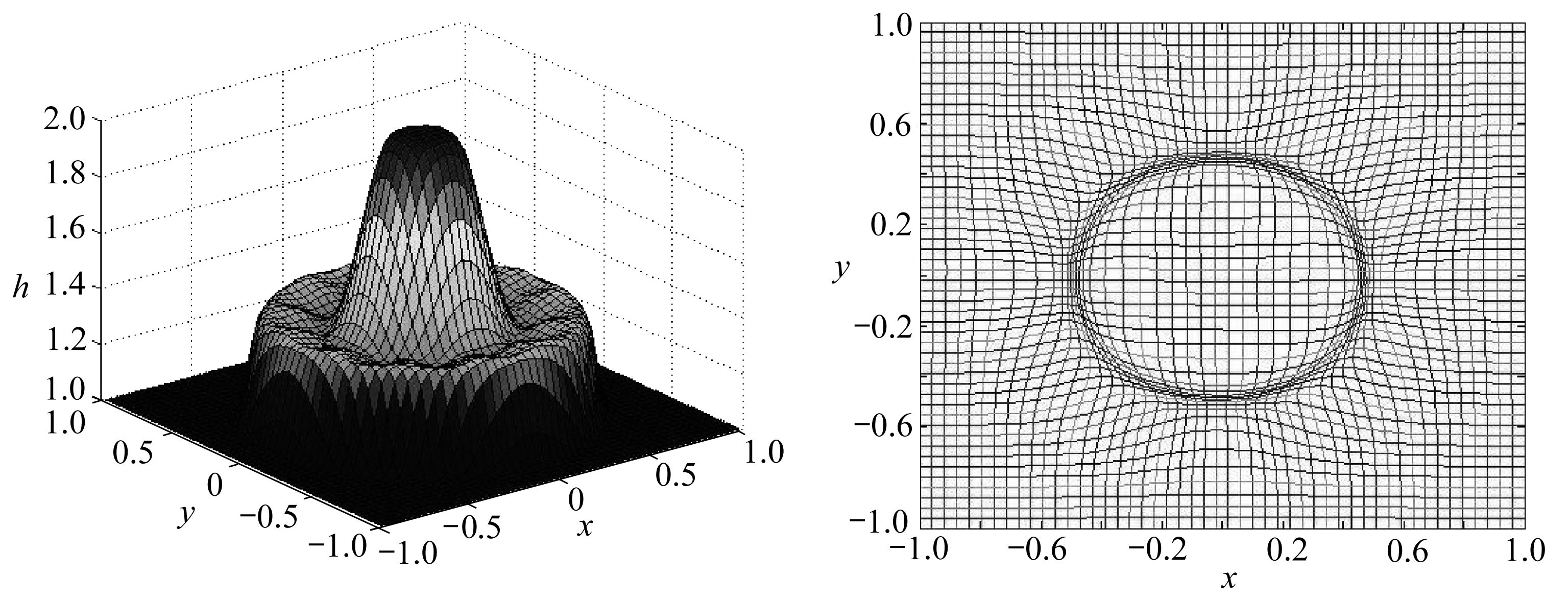Fig. 9 The moving grid circular dam simulation diagramFig. 10 The grid evolution map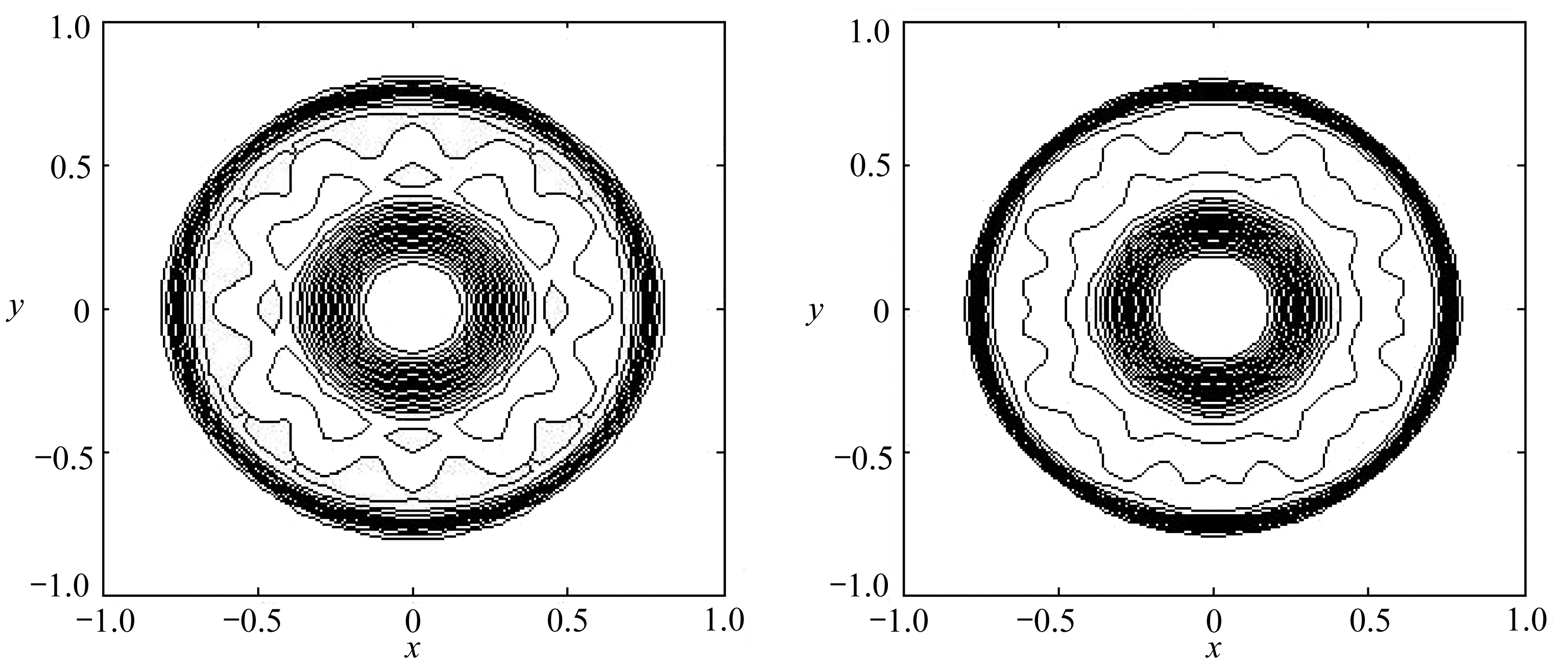(a) 固定网格结果 (b) 移动网格结果 (a) Fixed grid results (b) Moving grid results

Fig. 11 The water depth contours of the 2D circular dam breach problem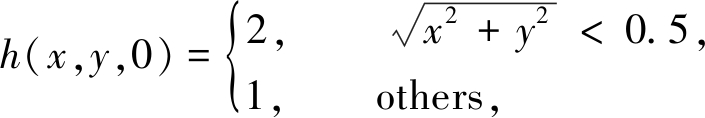u(x,y,0)=v(x,y,0)=0， zb(x,y)=0.

## 5 总 结

 TADMOR E. Entropy stability theory for difference approximations of nonlinear conservation laws and related time-dependent problems[J]. Acta Numerica, 2003, 12(12): 451-512.

 ISMAIL F, ROE P L. Affordable, entropy-consistent Euler flux functions Ⅱ: entropy production at shocks[J]. Journal of Computational Physics, 2009, 228(15): 5410-5436.

 FJORDHOLM U, MISHRAS, TADMOR E. Arbitrarily high-order accurate entropy stable essentially nonoscillatory schemes for systems of conservation laws[J]. SIAM Journal on Numerical Analysis, 2012, 50(2): 544-573.

 郑素佩, 封建湖, 刘彩侠. 高分辨率熵相容算法在二维溃坝问题中的应用[J]. 水动力学研究与进展(A辑), 2013, 28(5): 545-551.(ZHENG Supei, FENG Jianhu, LIU Caixia. Application of high resolution entropy compatible algorithm in two-dimensional dam failure problem[J]. Journal of Hydrodynamics, 2013, 28(5): 545-551.(in Chinese))

 CHENG X H, NIE Y F, FENG J H. Self-adjusting entropy-stable scheme for compressible Euler equations[J]. Chinese Physics B, 2015, 24(2): 020202. DOI: 10.1088/1674-1056/24/2/020202.

 RAY D, CHANDRASHEKAR P, FJORDHOLM U S. Entropy stable scheme on two-dimensional unstructured grids for Euler equations[J]. Communications in Computational Physics, 2016, 19(5): 1111-1140.

 张海军, 封建湖, 程晓晗. 带源项浅水波方程的高分辨率熵稳定格式[J]. 应用数学和力学, 2018, 39(8): 935-945.(ZHANG Haijun, FENG Jianhu, CHENG Xiaohan. An entropy stable scheme for shallow water equations with source terms[J]. Applied Mathematics and Mechanics, 2018, 39(8): 935-945.(in Chinese))

 HARTEN A, HYMAN J M. Self-adjusting grid methods for one-dimensional hyperbolic conservation laws[J]. Journal of Computational Physics, 1983, 50(2): 235-269.

 XU X H, NI G X. A high-order moving mesh kinetic scheme based on WENO reconstruction for compressible flows[J]. Journal of Scientific Computing, 2013, 27(2): 278-299.

 HAN E, LI J, TANG H. An adaptive GRP scheme for compressible fluid flows[J]. Journal of Computational Physics, 2010, 229(5): 1448-1466.

 CAO W, HUANG W, RUSSELL D. A study of monitor functions for two-dimensional adaptive mesh generation[J]. SIAM Journal on Scientific Computing, 1999, 20: 1978-1994.

 LI R, TANG T, ZHANG P. Moving mesh methods in multiple dimensions based on harmonic maps[J]. Journal of Computational Physics, 2001, 170(2): 562-588.

 TANG Z H, TANG T. Adaptive mesh method one- and two-dimensional hyperbolic conservation laws[J]. SIAM Journal on Numerical Analysis, 2003, 41(2): 487-515.

 杨继明, 陈艳萍. 一类奇异摄动对流扩散边值问题的移动网格方法[J]. 湘潭大学自然科学学报, 2004, 26(3): 24-29.(YANG Jiming, CHEN Yanping. A moving mesh method for a class of singularly perturbed convection-diffusion boundary value problems[J]. Journal of Natural Science of Xiangtan University, 2004, 26(3): 24-29.(in Chinese))

 陈冬冬, 宋松和. 变形法移动网格求解双曲型守恒律方程研究[J]. 计算机技术与发展, 2010, 20(2): 1-4.(CHEN Dongdong, SONG Songhe. Research of moving mesh method based on deformation in solving hyperbolic conservation laws[J]. Computer Technology and Development, 2010, 20(2): 1-4.(in Chinese))

 HE P, TANG H. An adaptive moving mesh method for two-dimensional relativistic hydrodynamics[J]. Communications in Computational Physics, 2012, 11(1): 114-146.

 程晓晗, 聂玉峰, 蔡力. 基于移动网格的熵稳定格式[J]. 计算物理, 2017, 34(2): 175-182.(CHENG Xiaohan, NIE Yufeng, CAI Li. Entropy stable scheme based on moving meshes for hyperbolic conservation laws[J]. Chinese Journal of Computational Physics, 2017, 34(2): 175-182.(in Chinese))

 BRADFORD S F, SANDERS B F. Finite-volume model for shallow-water flooding of arbitrary topography[J]. Journal of Hydraulic Engineering, 2002, 128(3): 289-298.

 BRUFAU P, GARC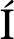A-NAVARRO M E. Zero mass error using unsteady wetting-drying conditions in shallow flows over dry irregular topography[J]. International Journal for Numerical Methods in Fluids, 2004, 45(10): 1047-1082.

 THANH M D, KARIM M F, ISMAIL A I M. Well-balanced scheme for shallow water equations with arbitrary topography[J]. International Journal of Dynamical Systems & Differential Equations, 2008, 1(3): 196-204.

 GOTTLIEB S, KETCHESON D I, SHU C W. High order strong stability preserving time discretizations[J]. Journal of Scientific Computing, 2009, 38(3): 251-289.

 朱华君. 二维浅水波方程的高阶有限体积法[D]. 硕士学位论文. 长沙: 国防科学技术大学, 2006.(ZHU Huajun. High-order finite volume method for two-dimensional shallow water wave equation[D]. Master Thesis. Changsha: National University of Defense Technology, 2006.(in Chinese))

 刘刚, 金生. 基于修正Roe格式的有限体积法求解二维浅水方程[J]. 水利水运工程学报, 2009(3): 29-33.(LIU Gang, JIN Sheng. Finite volume model for the 2D shallow water equations using modified Roe scheme[J]. Hydro-Science and Engineering, 2009(3): 29-33.(in Chinese))

 潘存鸿. 三角形网格下求解二维浅水方程的和谐Godunov格式[J]. 水科学进展, 2007, 18(2): 204-209.(PAN Cunhong. Well-balanced Godunov-type scheme for 2D shallow water flow with triangle mesh[J]. Advances in Water Science, 2007, 18(2): 204-209.(in Chinese))

 WINTERS A R, GASSNER G J. An entropy stable finite volume scheme for the equations of shallow water magnetohydrodynamics[J]. Journal of Scientific Computing, 2016, 67(2): 514-539.

 TORO E F. Riemann Solvers and Numerical Methods for Fluid Dynamics[M]. Berlin: Springer, 2013.

 王如云. 浅水波数值模拟的Roe平均法[J]. 计算物理, 2000, 17(2): 199-203.(WANG Ruyun. Roe mean method for numerical simulation of shallow water waves[J]. Chinese Journal of Computational Physics, 2000, 17(2): 199-203.(in Chinese))

 AZARENOK B N. Variational method for adaptive mesh generation[J]. Computational Mathematics and Mathematical Physics, 2008, 48(5): 786-804.

 EELLS J, SAMPSON J H. Harmonic mappings of Riemannian manifolds[J]. American Journal of Mathematics, 1964, 86(1): 109-160.

# Solution of 2D Shallow Water Wave Equations With the Moving-Grid Rotating-Invariance Method

ZHENG Supei, WANG Ling, WANG Miaomiao

(School of Sciences, Changan University, Xian 710064, P.R.China)

Abstract: In order to improve the resolution of the numerical algorithm for solving the 2D shallow water wave equation, a new algorithm was proposed based on the moving-grid method, with the entropy stable numerical flux function and by means of the mixed numerical flux obtained through the rotating invariance. The numerical solution of the shallow water wave equation and the grid computation process based on the characteristics of the solution were interleaved. The variational principle was used to reconstruct the mesh, and the physical quantity on the new mesh was computed with the 2nd-order precision conservation interpolation formula. The 3rd-order strongly stable Runge-Kutta method and the entropy stable format satisfying the 2nd law of thermodynamics were used to numerically solve the shallow water wave equation. The numerical results show that, the new algorithm has good discontinuity capture ability and high resolution.

Key words: moving-grid method; rotating invariance; entropy stable format; Runge-Kutta method; finite volume method

ⓒ 应用数学和力学编委会，ISSN 1000-0887

http://www.applmathmech.cn

*收稿日期： 2019-03-26；

DOI: 10.21656/1000-0887.400124

Foundation item: The National Natural Science Foundation of China(11401045; 11971075)